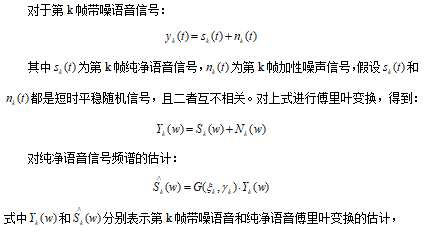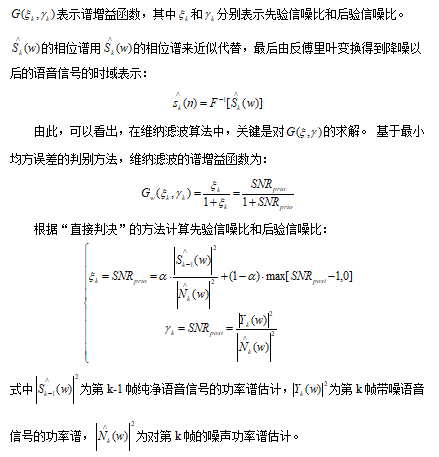# 【语音去噪】基于基本维纳滤波算法语音去噪matlab源码

• 时间:
• 浏览:

## 一、简介

1、语音增强概述
1.1 语音增强的相关概念

1.2 语音增强的相关算法

2 基于先验信噪比估计的维纳滤波语音增强理论## 二、源代码

``````clear all; clc; close all;

[xx, fs] = wavread('C5_3_y.wav');           % 读入数据文件
xx=xx-mean(xx);                         % 消除直流分量
x=xx/max(abs(xx));                      % 幅值归一化
IS=0.25;                                % 设置前导无话段长度
wlen=200;                               % 设置帧长为25ms
inc=80;                                 % 设置帧移为10ms
SNR=5;                                  % 设置信噪比SNR
NIS=fix((IS*fs-wlen)/inc +1);           % 求前导无话段帧数
alpha=2;
beta=0.01;
signal=awgn(x,SNR,'measured','db');               % 叠加噪声
output=Weina_Norm(x,wlen,inc,NIS,alpha,beta) ;
output=output/max(abs(output));
len=min(length(output),length(x));
x=x(1:len);
signal=signal(1:len);
output=output(1:len);

snr1=SNR_Calc(x,signal);            % 计算初始信噪比
snr2=SNR_Calc(x,output);            % 计算降噪后的信噪比
snr=snr2-snr1;
fprintf('snr1=%5.4f   snr2=%5.4f   snr=%5.4f\n',snr1,snr2,snr);
function frameout=enframe(x,win,inc)

nx=length(x(:));            % 取数据长度
nwin=length(win);           % 取窗长
if (nwin == 1)              % 判断窗长是否为1，若为1，即表示没有设窗函数
len = win;               % 是，帧长=win
else
len = nwin;              % 否，帧长=窗长
end
if (nargin < 3)             % 如果只有两个参数，设帧inc=帧长
inc = len;
end
nf = fix((nx-len+inc)/inc); % 计算帧数
frameout=zeros(nf,len);            % 初始化
indf= inc*(0:(nf-1)).';     % 设置每帧在x中的位移量位置
inds = (1:len);             % 每帧数据对应1:len
frameout(:) = x(indf(:,ones(1,len))+inds(ones(nf,1),:));   % 对数据分帧
if (nwin > 1)               % 若参数中包括窗函数，把每帧乘以窗函数
w = win(:)';            % 把win转成行数据
function frameout=filpframe(x,win,inc)

[nf,len]=size(x);
nx=(nf-1) *inc+len;                 %原信号长度
frameout=zeros(nx,1);
nwin=length(win);                   % 取窗长
if (nwin ~= 1)                           % 判断窗长是否为1，若为1，即表示没有设窗函数
winx=repmat(win',nf,1);
x=x./winx;                          % 除去加窗的影响
x(find(isinf(x)))=0;                %去除除0得到的Inf
end

sig=zeros((nf-1)*inc+len,1);
for i=1:nf
start=(i-1)*inc+1;
xn=x(i,:)';
sig(start:start+len-1)=sig(start:start+len-1)+xn;
end

% 设置缺省值
if nargin<4
NoiseMargin=3;
end
if nargin<5
Hangover=8;
end
if nargin<3
NoiseCounter=0;
end

% 本帧语音幅值对数频谱和噪声对数频谱之差值
SpectralDist= 20*(log10(signal)-log10(noise));
SpectralDist(find(SpectralDist<0))=0;   % 寻找差值小于0值置为0

Dist=mean(SpectralDist);                % 用平均求出Dist
if (Dist < NoiseMargin)                 % Dist 是否小于 NoiseMargin
NoiseFlag=1;                        % 是，NoiseFlag设为1
NoiseCounter=NoiseCounter+1;        % NoiseCounter加1
else
NoiseFlag=0;                        % 否，NoiseFlag设为0
NoiseCounter=0;                     % NoiseCounter清零
end
``````

## 三、运行结果